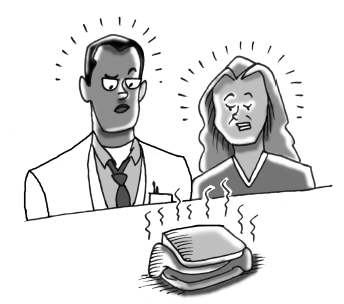### Home > CCA2 > Chapter Ch3 > Lesson 3.2.3 > Problem3-94

3-94.Janelle conducted an experiment by mistake when she left her bologna sandwich at school over winter break. When she got back, her sandwich was much larger than it was when she left it. Her science teacher explained that the sandwich had produced large quantities of a rare bacterium, Bolognicus sandwichae. Based on a sample taken from the sandwich, Janelle determined that there were approximately $72$ million bacteria present. Her science teacher explained that this is not very surprising, since the number of this bacteria triples every $24$ hours. Since the sandwich had been made only $15$ days ago, Janelle was sure that she could sue the meat company. The food industry standard for the most bacteria a sandwich-sized portion can have at the time of production is $100$. Find out how many of the bacteria were present when the sandwich was made to determine if Janelle has a case. Homework Help ✎

Use the equation $y=ab^x$.

$b=\text{multiplier}=3$
$a=\text{starting point (today)}=72\text{ million}$
What exponent should you use for $15$ days before today?

If you aren't sure, think about a simpler problem: what would you use for yesterday?
The day before that? Notice that $x=1$ and $x=2$ would represent tomorrow and the day after that.